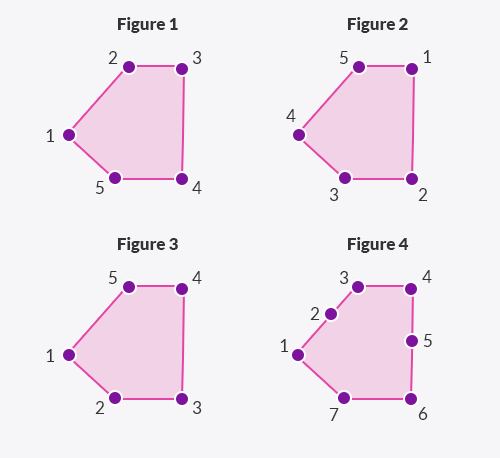Deals Of The Week - hours only!Up to 80% off on all courses and bundles.-Close
Introduction
ST_Equals
4. How ST_Equals works
Relationships Between Geometries
ST_Distance and ST_Dwithin
Spatial joins practice
Summary

## Instruction

Nicely done! As we've already mentioned, ST_Equals checks whether two geometries represent the same location or space. ST_Equals ignores the order of vertices in a polygon, the starting point of a polygon, and even additional vertices that do not change its shape. Look at the image below:ST_Equals will consider Figure 1, Figure 2, Figure 3, and Figure 4 to be equal, even though their representations are certainly different. Take a look at the following query:

SELECT *
FROM sf_hotels sh
JOIN sf_sights ss
ON ST_Equals(sh.boundaries,
ST_Reverse(ss.coordinates))


As you can see, we passed in the ST_Reverse function as one of the arguments to ST_Equals. The ST_Reverse function returns a geometry with the vertex order reversed. So, if we had the linestring ABC, the ST_Reverse function would return the linestring CBA.

In accordance with our earlier explanation, even though we reversed the vertex order of one of its arguments, the ST_Equals function still returned TRUE and gave us the same result.

## Exercise

Click the button and note that both ST_Equals usages return the same result.